## 20 Questions MCQ Test GATE ECE (Electronics) 2023 Mock Test Series | Test: Spread Spectrum

Description
Attempt Test: Spread Spectrum | 20 questions in 60 minutes | Mock test for Electronics and Communication Engineering (ECE) preparation | Free important questions MCQ to study GATE ECE (Electronics) 2023 Mock Test Series for Electronics and Communication Engineering (ECE) Exam | Download free PDF with solutions
QUESTION: 1

### A rate 1/2 convolution code with dfrec = 10 is used to encode a data requeence occurring at a rate of 1 kbps. The modulation is binary PSK. The DS spread spectrum sequence has a chip rate of 10 MHz Que: The coding gain is

Solution: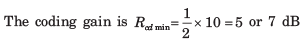QUESTION: 2

### A rate 1/2 convolution code with dfrec = 10 is used to encode a data requeence occurring at a rate of 1 kbps. The modulation is binary PSK. The DS spread spectrum sequence has a chip rate of 10 MHz Que: The processing gain is

Solution:

The processing gainQUESTION: 3

### A CDMA system is designed based on DS spread spectrum with a processing gain of 1000 and BPSK modulation scheme. If user has equal power and the desired level of performance of an error probability of 10-6, the number of user will be

Solution:

To achieve an error probability of 10-6 ,we required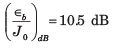Then, the number of users of the CDMA system is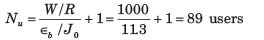QUESTION: 4

In previous question if processing gain is changed to 500, then number of users will be

Solution:

If the processing gain is reduced to W/R = 500, then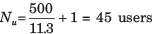QUESTION: 5

A DS spread spectrum system transmit at a rate of 1 kbps in the presets of a tone jammer. The jammer power is 20 dB greater then the desired signal, and the required εb/J0 to achieve satisfactory performance is 10 dB.

Que: The spreading bandwidth required to meet the specifications is

Solution:

We have a system where  (Jav/Pav) = 20 dB, R =1000, and (εb/J0)db = 10 dB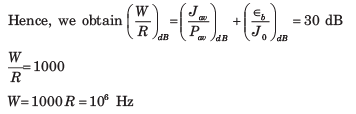QUESTION: 6

A DS spread spectrum system transmit at a rate of 1 kbps in the presets of a tone jammer. The jammer power is 20 dB greater then the desired signal, and the required εb/J0 to achieve satisfactory performance is 10 dB.

Que: If the jammer is a pulse jammer, then pulse duty cycle that results in worst case jamming is

Solution:

The duty cycle of a pulse jammer of worst-case jamming is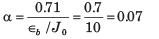QUESTION: 7

A DS spread spectrum system transmit at a rate of 1 kbps in the presets of a tone jammer. The jammer power is 20 dB greater then the desired signal, and the required εb/J0 to achieve satisfactory performance is 10 dB.

Que: The correspond probability of error is

Solution:

The corresponding probability of error for this worst-case jamming is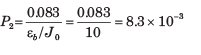QUESTION: 8

A CDMA system consist of 15 equal power user that transmit information at a rate of 10 kbps, each using a DS spread spectrum signal operating at chip rate of 1 MHz. The modulation scheme is BPSK

Que: The Processing gain is

Solution: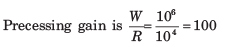QUESTION: 9

A CDMA system consist of 15 equal power user that transmit information at a rate of 10 kbps, each using a DS spread spectrum signal operating at chip rate of 1 MHz. The modulation scheme is BPSK

Que: The value of εb/Jb is

Solution:

We have N= 15 users transmitting at a rate of 10,000 bps each, in a bandwidth of W =1MHz.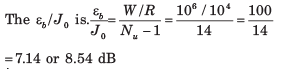QUESTION: 10

A CDMA system consist of 15 equal power user that transmit information at a rate of 10 kbps, each using a DS spread spectrum signal operating at chip rate of 1 MHz. The modulation scheme is BPSK

Que: How much should the processing gain be increased to allow for doubling the number of users with affecting the autopad SNR

Solution: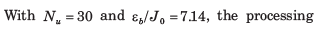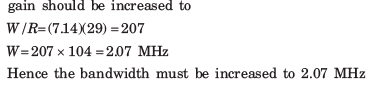QUESTION: 11

An m =10 ML shift register is used to generate the pre hdarandlm sequence in a DS spread spectrum system. The chip duration is Tc = ℓμs  and the bit duration is Tb, = NTc, where N is the length (period of the m sequence).

Que: The processing gain of the system is

Solution:

The period of the maximum length shift register sequence is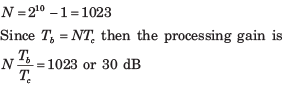QUESTION: 12

An m =10 ML shift register is used to generate the pre hdarandlm sequence in a DS spread spectrum system. The chip duration is Tc = ℓμs  and the bit duration is Tb, = NTc, where N is the length (period of the m sequence).

Que: If the required ε/J0 is 10 and the jammer is a tone jammer with an average power Jav, then jamming margin is.

Solution:

A Jamming margin is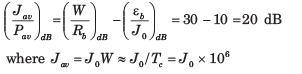QUESTION: 13

An FH binary orthogonal FSK system employs an m = 15 stage liner feedback shift register that generates an ML sequence. Each state of the shift register selects one of L non over lapping frequency bands in the hopping pattern. The bit rate is 100 bits/s. The demodulator employ non coherent detection.

Que: If the hop rate is one per bit, the hopping band width for this channel is

Solution:

The length of the shift-register sequence is

L = 2m - 1215 - 1 = 32767 bits

For binary FSK modulation, the minimum frequency separation is 2/T, where 1/T is the symbol (bit) rate.
The hop rate is 100 hops/sec. Since the shift register has L = 32767 states and each state utilizes a bandwidth of 2/T = 200 Hz, then the total bandwidth for the FH signal is 6.5534 MHz.

QUESTION: 14

An FH binary orthogonal FSK system employs an m = 15 stage liner feedback shift register that generates an ML sequence. Each state of the shift register selects one of L non over lapping frequency bands in the hopping pattern. The bit rate is 100 bits/s. The demodulator employ non coherent detection

Que: Suppose the hop rate is increased to 2 hops/bit and the receiver uses square law combining the signal over two hops. The hopping bandwidth for this channel is

Solution:

If the hopping rate is 2 hops/bit and the bit rate is 100 bits/sec, then, the hop rate is 200 hops/sec. The minimum frequency separation for orthogonality 2/T =400 Hz. Since there are N = 2767 states of the shift register and for each state we select one of two frequencies separated by 400 Hz, the hopping bandwidth is 13.1068 MHz.

QUESTION: 15

In a fast FH spread spectrum system, the information is transmitted via FSK with non coherent detection. Suppose there are N = 3 hops/bit with hard decision decoding of the signal in each hop. The channel is AWGN with power spectral density 1/2 N0 and an SNR 20-13 dB (total SNR over the three hops)

Que: The probability of error for this system is

Solution:

The total SNR for three hops is 20 ~ 13 dB. Therefore the SNR per hop is 20/3. The probability of a chip error with non-coherent detection is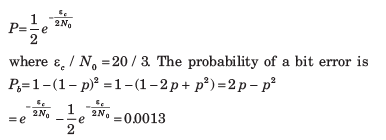QUESTION: 16

In a fast FH spread spectrum system, the information is transmitted via FSK with non coherent detection. Suppose there are N = 3 hops/bit with hard decision decoding of the signal in each hop. The channel is AWGN with power spectral density 1/2 N0 and an SNR 20-13 dB (total SNR over the three hops)

Que: In case of one hop per bit the probability of error is

Solution:

In the case of one hop per bit, the SNR per bit is 20,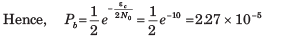QUESTION: 17

A slow FH binary FSK system with non coherent detection operates at ε0/J0 10, with hopping bandwidth of 2 GHz, and a bit rate of 10 kbps.

Que: The processing gain of this system is

Solution:

We are given a hopping bandwidth of 2 GHz and a bit rate of 10 kbs.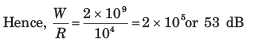QUESTION: 18

A slow FH binary FSK system with non coherent detection operates at ε0/J0 10, with hopping bandwidth of 2 GHz, and a bit rate of 10 kbps.

Que: If the jammer operates as a partial band jammar, the bandwidth occupancy for worst case jamming is

Solution:

The bandwidth of the worst partial-band jammer is α * W , where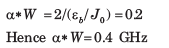QUESTION: 19

A slow FH binary FSK system with non coherent detection operates at ε0/J0 10, with hopping bandwidth of 2 GHz, and a bit rate of 10 kbps.

Que: The probability of error for the worst-case partial band jammer is

Solution:

The probability of error with worst-case partial-band jamming is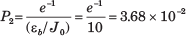QUESTION: 20

A slow FH binary FSK system with non coherent detection operates at ε0/J0 10, with hopping bandwidth of 2 GHz, and a bit rate of 10 kbps.

Que: The minimum hop rate for a FH spread spectrum system that will prevent a jammer from operating five onives away from the receiver is

Solution:

d = 5 miles = 8050 meters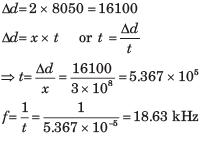Use Code STAYHOME200 and get INR 200 additional OFF Use Coupon Code